# GRE Subject Test: Math : Order of Convergence

## Example Questions

### Example Question #1 : Numerical Approximation

For which values of p is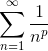convergent?

All positive values of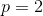only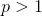it doesn't converge for any values ofonlyExplanation:

We can solve this problem quite simply with the integral test. We know that if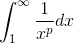converges, then our series converges.

We can rewrite the integral as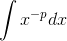and then use our formula for the antiderivative of power functions to get that the integral equals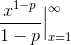.

We know that this only goes to zero if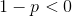. Subtracting p from both sides, we get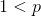.

### All GRE Subject Test: Math Resources